#The Model Student: A Rube-ish Square (Part 1)

Overload Journal #89 - February 2009 + Design of applications and programs   Author: Richard Harris
We all have nostalgia for favourite childhood toys. Richard Harris looks at the maths behind a classic.

For those of us of a certain age, the creak-creak-creak of an orchestra of Rubik's Cubes [Rubiks] provided the soundtrack to many a childhood lunch break. There are no doubt a few amongst us who were elevated from socially awkward outsiders to school yard super heroes after having learnt the Secrets of the Cube Masters [Taylor81] . You may be surprised to read that I was not one of this number. Socially awkward yes, but I never learnt how to solve that fiendish puzzle.

What I, and I suspect many of us, did not realise at the time was that Rubik's Cube is far more than just a toy. It is in fact a physical manifestation of the mathematical subject of group theory.

Group theory can be thought of as the mathematics of symmetry. It was to group theory that I turned when considering the number of tours in the regular travelling salesman problem [Harris07] , although I didn't discuss it in those terms in the article. In the case of Rubik's Cube, each rotational manipulation leaves the cube in a state symmetric to the previous; namely a cube. The coloured stickers are simply labels to inform us of which of these symmetric states the cube is currently in.

Rubik's Cube has a staggering number of states; 43,252,003,274,489,856,000 all told [Rubiks] . Indeed, there are so many that the original manufacturer downplayed the figure because they thought that the public wouldn't believe it [Rubiks2] . It is still an open question as to what is the largest number of moves required to return the cube to its initial state from any other state, with the latest estimate being 26 [Kunkle07] .

Because of this complexity, I propose that rather than analysing Rubik's Cube itself, we should study a simpler, analogous, problem. That problem is a two-dimensional version of the cube, which I shall call the Rube-ish Square.

The Rube-ish Square is defined as a three by three grid containing the numbers one to nine. Each row and column can be rotated left or right and up or down respectively with the number being pushed out of the grid returning on the opposite side, as illustrated in figure 1.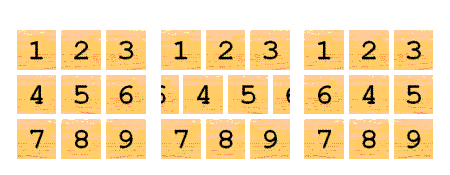Figure 1

## The joy of group ... theory

In order to understand the properties of this puzzle we must determine what kind of group it represents, and in order to do that we must first understand what a group actually is.

A group is defined by a set of elements and an associative (i.e. independent of the order of application) binary operator (usually denoted by ) that uniquely maps a pair of elements from the set onto a third, also from the set. Furthermore, there must be a unique identity element, i, that when combined via the operator with any other element of the set results in that element. Finally, for each element of the set, x, there must be a unique inverse element, denoted x-1, such that when they are both combined, the identity element results. Formally, for a group G this can be expressed as:

 Closed: ∀a, b ∈ G ⇒ a ◦ b ∈ G Associative: ∀a, b, c ∈ G ⇒ a ◦ (b ◦ c) = (a ◦ b) ◦ c Identity: ∃i ∈ G such that ∀a ∈ G a ◦ i = i ◦ a = a Inverse: ∀ a ∈ G ⇒ ∃a-1 ∈ G such that a ◦ a-1 = a-1 ◦ a = i

Now, I understand that this definition is rather loaded with mathematical jargon. To clarify, the upside down A means for all, the backwards E means there exists and the rounded E means within.

Translating, these rules mean that for a group G:

 Closed: For all a and b within G, a ◦ b is within G Associative: For all a, b and c within G, a ◦ (b ◦ c) = (a ◦ b) ◦ c Identity: There exists a unique element i within G such that for all a within G, a ◦ i = i ◦ a = a Inverse: For all a within G, there exists a unique element a-1 within G such that a ◦ a-1 = a-1 ◦ a = i

I suspect that this may not have entirely cleared up matters, so let's look at a specific example; modular arithmetic. Modular arithmetic, otherwise known as clock arithmetic, is defined as the addition of non-negative integers less than a given upper bound with the rule that when a result is equal to or greater than the upper bound, the upper bound is subtracted from it to return it to a number less than the upper bound.

Specifically, if we have an upper bound of n and a and b both less than n (and hence members of our group):

 If a + b < n then a ◦ b = a + b Else a ◦ b = a + b - n

This rule ensures that the result of the addition must be greater than or equal to zero and less than n and hence within the group. Since addition is associative, our operator must also be associative. The number zero acts as the identity and for any integer a less than n, the number n - a acts as the inverse, yielding the identity after addition under this rule.

If we choose n to be twelve, we can see why this is also known as clock arithmetic. Working with hours on a twelve hour clock and counting from the previous twelve o'clock we have, for example:

 Closed: 5 hours + 9 hours = 2 hours Associative: 5 hours + (6 hours + 3 hours) = 2 hours= (5 hours + 6 hours) + 3 hours Identity: 5 hours + 0 hours = 5 hours Inverse: 5 hours + 7 hours = 0 hours

Whilst it is clear that clock arithmetic follows the rules required for a group, it is perhaps not so obvious what this has to do with the mathematics of symmetry that I asserted was at the heart of group theory.

The symmetry of clock arithmetic reveals itself if we connect the numerals with straight lines to form a regular twelve sided polygon. Now, instead of moving the hour hand by a given number of hours, we keep it pointing up and rotate the clock beneath it, as illustrated in figure 2.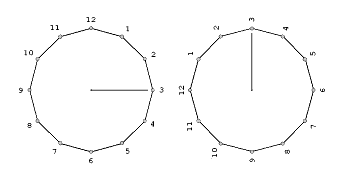Figure 2

Each rotation yields an identical, albeit relabelled, polygon, showing that the clock arithmetic captures the rotational symmetry of the dodecagon.

So, now that we have described what groups are, let's take a look at some of their properties.

Firstly, we shall show that my requirement that the identity be unique was superfluous. To see why, let's assume that there are two identities for a given group, i and i´. Consider:

i´ ◦ i

Now, by the rule for identities, this implies both:

i´ ◦ a = ai´ ◦ i = i

and:

ai = ai´ ◦ i = i´

and hence:

i´ = i

So the rule describing identities ensures that they must be unique for any given group.

Secondly, the requirement that the inverse of an element of a group is unique is also redundant. Assuming two inverses of the element a, a-1 and a*, we have by the rule for inverses:

 a-1 ◦ a = i a ◦ a* = i

Now the associative rule further implies:

 (a-1 ◦ a) ◦ a* = a-1 ◦ (a ◦ a*) i ◦ a* = a-1 ◦ i

and so by the identity rule we have:

a* = a-1

proving that each element can have only one inverse.

## Groups within groups within groups man

An important concept in group theory is that of the subgroup. A subgroup is comprised of a subset of the elements of a group that within themselves conform to the rules governing groups. The mathematical notation to indicate that a group H is a subgroup of a group G is:

HG

As an example of a subgroup, let's again take the clock arithmetic but this time only with the even-numbered hours. To show that this forms a group, consider each of the rules.

• Since the sum of two even numbers is always even and subtracting twelve from an even number greater than or equal to twelve also results in an even number, this set of numbers is closed under addition modulo twelve.
• We are still dealing with addition, so associativity is a given.
• Zero is an even number, so we have an identity element.
• Subtracting an even number less than twelve from twelve results in an even number less than twelve, so we have an inverse for every number in the set.

Hence the even numbered hours in the clock arithmetic form a subgroup of the clock arithmetic.

The clock arithmetic group has an important additional property that it shares with many, but not all, groups; that of commutativity. Translating into English, this means that the order in which the elements of the group are presented to the operator is irrelevant; that . Such groups are known as abelian groups and this additional property is formally expressed as:

Communtative: ∀ a, bGab = ba

Examples of groups that do not share this property are those of the permutation groups. Permutation groups describe the properties of reordering sets of elements and formed the original definition of groups when group theory was first developed in the early nineteenth century [Baumslag68] .

To informally show that permutations can form groups we again consider the rules defining groups.

• If we reorder a set of elements and then reorder it again we trivially have another reordering of the elements and hence permutations are closed.
• If we reorder the elements once and then, considered together, a twice and third time we will have the same permutation that we would have had if we had instead reordered them with the first and second permutations considered together and then the third permutation and hence they are associative.
• We can leave the elements in the order we found them, so they have an identity element.
• Finally, we can sort the elements back into their original order, so each permutation has an inverse.

The set of all possible permutations of a set of n elements is known as the symmetric group of degree n, or Sn. The usual notation for a permutation is two rows of numbers, the first listing the positions of elements before they are reordered and the second listing their positions after they are reordered. For example, one permutation of a set of three elements is:Applied to the set of elements (a, b, c) this results in (b, c, a). The first element becomes the third, the second becomes the first and the third becomes the second. Applying it again would yield (c, a, b), or the permutation: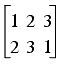Applying it a third time results in (a, b, c), giving us the identity permutation: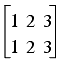The symmetric group of degree three has the following six elements: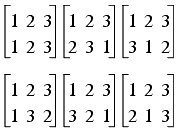We can show that this group is not abelian by taking a pair of permutations and applying them in both orders:Note that I've adopted the convention that the permutation on the left hand side of the operator is applied to the set first and the permutation on the right hand side second. The opposite convention, with the permutation on the right hand side applied first, would also describe a group, albeit one in which combining pairs of elements would yield different results.

The first row of elements in the full group above contains the three permutations first described. These are the permutations that swap two pairs of elements whilst the second row contains those permutations that result swap just one pair.

In a similar fashion to the even-numbered hours of the clock arithmetic, permutations with an even number of swapped pairs form a subgroup of a symmetric group, known as the alternating group of degree n, or An.

Calling the first permutation described above p, we have already shown that applying it three times results in the identity, so:

ppp = p3 = i

and hence:

pp = p2 = p-1

giving us inverses for both p and p2, thus confirming that this is indeed a group.

The permutation p is known as a generator of the group since by repeatedly applying it we generate every element of the group. Formally, a generator of a group is a set of elements that, together with their inverses, yield every element of the group through repeated application of the operator.

## Ladies and gentlemen: the point!

So now that we are familiar with the basic properties of groups we are ready to investigate the properties of the Rube-ish Square. The first step is to determine which group captures its symmetries. We can begin by noting that each rotation of a row or column results in different permutation of the squares, so we can represent it using the permutation notation.

By definition the rotations of the rows to their left, the rotations of the columns upwards and their inverse rotations must, by repeated application, generate every possible state of the square since they are the only operations with which we can manipulate it and hence are a generator for our group. We name these r1, r2, r3 and c1, c2, c3 as illustrated in figure 3.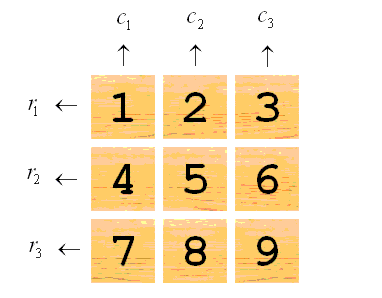Figure 3

These are represented as resulting states, in permutation notation and by exchanges of elements as follows: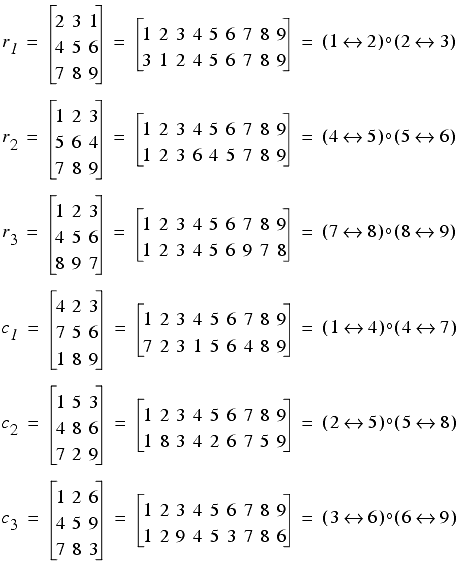The first thing that we should note is that all of these are even permutations since they each swap two pairs of elements of the square. Our Rube-ish Square must therefore be described by either the alternating group of degree nine, or a subgroup of it.

The question as to whether the Rube-ish Square is described by the alternating group is equivalent to the question as to whether any two pairs of elements of the square can be exchanged using a combination of elements from the generator set. We can answer that question by examining permutations of the form:

aba-1

and, since an element is by definition the inverse of its inverse, also of the form:

a-1ba

Specifically, we're interested in four of these permutations: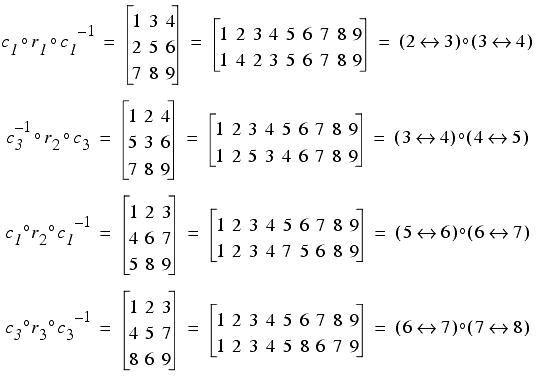By chaining these and r1, r2 and r3 together we can exchange any pair of two sequentially adjacent elements of the Rube-ish Square. For example, to exchange element 2 and 3 and 5 and 6, we apply the chain:

 (c1 ◦ r1 ◦ c1-1) ◦ (c3-1 ◦ r2 ◦ c3) ◦ (r2) = (2 ↔ 3) ◦ (3 ↔ 4) ◦ (3 ↔ 4) ◦ (4 ↔ 5) ◦ (4 ↔ 5) ◦ (5 ↔ 6) = (2 ↔ 3) ◦ (3 ↔ 4) ◦ (3 ↔ 4) ◦ (4 ↔ 5) ◦ (4 ↔ 5) ◦ (5 ↔ 6) = (2 ↔ 3) ◦ (5 ↔ 6)

We can also manipulate the square itself to confirm that this works: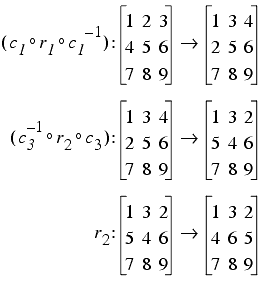The notation here is that of a mapping and indicates that the permutation on the left of the colon maps, or transforms, the state immediately to the right of it to the one following the arrow. As predicted, the elements 2 and 3 and 5 and 6 have been swapped by this permutation.

The next question is whether being able to exchange any two sequentially adjacent pairs of elements implies that we can exchange any two pairs of elements. To answer this, consider a chain of exchanges of sequentially adjacent pairs in which one pair appears at every step. For example:

(1 ↔ 2) ◦ (4 ↔ 5)
(1 ↔ 2) ◦ (5 ↔ 6)
(1 ↔ 2) ◦ (6 ↔ 7)

The effect of this permutation upon the state of the square is as follows:With this chain of permutations, we have moved the 4th element to the 7th position, keeping all the elements between the 4th and the 7th in their original order. We can move the original 7th element to the 4th position by applying a similar chain in the opposite direction: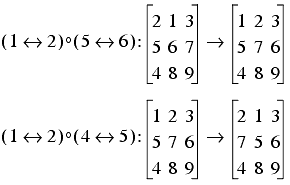with the result that we have swapped elements 1 and 2 and 4 and 7.

We could repeat the operation with another non-sequentially adjacent pair together with elements 1 and 2 to exchange two arbitrary pairs since the elements 1 and 2 would be swapped back to their original order.

Unfortunately, this scheme breaks down when we want to move either element 1 or element 2. To employ this approach in this situation we need simply note that there is nothing special about these elements; we could just as easily have chosen elements 8 and 9. As we march a given element up or down through the sequence, we should employ an adjacent pair that will not interfere with the intended step. As the moving element approaches the currently employed pair we can simply swap them with another pair since we have already shown that we can construct a permutation that swaps any two sequentially adjacent pairs of elements. For example, if we wish to swap elements 4 and 2 we could employ the following sequence of exchanges: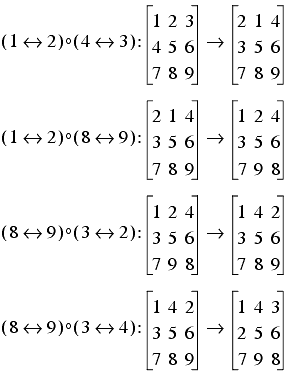Since we only move a single element within the sequence during each step, we can always find a sequentially adjacent pair to exploit that won't interfere with the element's journey. Hence we can construct a permutation that will exchange any two arbitrary pairs of elements using our generator set.

Therefore, the generator set for the Rube-ish Square can generate any even permutation of the elements of the square and so the group that describes it must be the alternating group of degree nine. This means that the number of states of the Rube-ish Square must be equal to the number of elements of the alternating group of degree nine, or:Now, I am well aware that this article has been rather maths heavy and for that you have my sincerest apologies. Thankfully, the next question I wish to raise about the Rube-ish Square is that of determining which state requires the most moves to return it to the initial state and, much as this is an unsolved question for Rubik's Cube, we will not be able to answer this mathematically. Next time, dear reader, there will be code.